SNOSBJ3E November   1999  – December 2014

PRODUCTION DATA.

1. Features
2. Applications
3. Description
4. Revision History
5. Pin Configuration and Functions
6. Specifications
7. Detailed Description
8. Application and Implementation
1. 8.1 Application Information
2. 8.2 Typical Applications
1. 8.2.1 Basic Comparator
2. 8.2.2 System Examples
9. Power Supply Recommendations
10. 10Layout
11. 11Device and Documentation Support
12. 12Mechanical, Packaging, and Orderable Information

• J|14

## 6 Specifications

### 6.1 Absolute Maximum Ratings(1)

MIN MAX UNIT
Supply Voltage, V+ LM139N, LM239N, LM339N, LM2901N 36 VDC
LM3302N 28
Differential Input Voltage LM139N, LM239N, LM339N, LM2901N (2) 36
LM3302N(2) 28
Input Voltage LM139N, LM239N, LM339N, LM2901N −0.3 36
LM3302 –0.3 28
Input Current (VIN<−0.3 VDC)(3) 50 mA
Power Dissipation(4) PDIP 1050 mW
Cavity DIP 1190
SOIC Package 760
Output Short-Circuit to GND(5) Continuous
Lead Temperature (Soldering, 10 seconds) 260 °C
Soldering Information PDIP Package (10 seconds) 260
SOIC Package Vapor Phase (60 seconds) 215
Infrared (15 seconds) 220
Storage temperature, Tstg −65 150
(1) Refer to RETS139AX for LM139A military specifications and to RETS139X for LM139 military specifications.
(2) Positive excursions of input voltage may exceed the power supply level. As long as the other voltage remains within the common-mode range, the comparator will provide a proper output state. The low input voltage state must not be less than −0.3 VDC (or 0.3 VDC below the magnitude of the negative power supply, if used) (at 25°C).
(3) This input current will only exist when the voltage at any of the input leads is driven negative. It is due to the collector-base junction of the input PNP transistors becoming forward biased and thereby acting as input diode clamps. In addition to this diode action, there is also lateral NPN parasitic transistor action on the IC chip. This transistor action can cause the output voltages of the comparators to go to the V+ voltage level (or to ground for a large overdrive) for the time duration that an input is driven negative. This is not destructive and normal output states will re-establish when the input voltage, which was negative, again returns to a value greater than −0.3 VDC (at 25°C).
(4) For operating at high temperatures, the LM339/LM339A, LM2901, LM3302 must be derated based on a 125°C maximum junction temperature and a thermal resistance of 95°C/W which applies for the device soldered in a printed circuit board, operating in a still air ambient. The LM239-N and LM139-N must be derated based on a 150°C maximum junction temperature. The low bias dissipation and the “ON-OFF” characteristic of the outputs keeps the chip dissipation very small (PD≤100 mW), provided the output transistors are allowed to saturate.
(5) Short circuits from the output to V+ can cause excessive heating and eventual destruction. When considering short circuits to ground, the maximum output current is approximately 20 mA independent of the magnitude of V+.

### 6.2 ESD Ratings

VALUE UNIT
V(ESD) Electrostatic discharge Human-body model (HBM), per ANSI/ESDA/JEDEC JS-001(1) ±600 V
(1) JEDEC document JEP155 states that 500-V HBM allows safe manufacturing with a standard ESD control process.

### 6.3 Recommended Operating Conditions

over operating free-air temperature range (unless otherwise noted)
MIN MAX UNIT
Supply Voltage Single Supply LM139N, LM239N, LM339N, LM2901N 2 36 V
LM3302N 2 28
Dual Supply LM139N, LM239N, LM339N, LM2901N ±1 ±18
LM3302N ±1 ±14
Operating Temperature LM139/LM139A −55 125 °C
LM2901/LM3302 −40 85
LM239/LM239A −25 85
LM339/LM339A 0 70

### 6.4 Thermal Information

THERMAL METRIC(1) LM139-N, LM239-N, LM339-N LM2901-N, LM339-N LM2901-N, LM339-N UNIT
J D NFF
14 PINS
RθJA Junction-to-ambient thermal resistance 95 95 95 °C/W

### 6.5 Electrical Characteristics: LM139A, LM239A, LM339A, LM139

(V+=5 VDC, TA = 25°C(1) unless otherwise stated)
PARAMETER TEST CONDITIONS LM139A LM239A, LM339A LM139 UNIT
MIN TYP MAX MIN TYP MAX MIN TYP MAX
Input Offset Voltage See(2) 1.0 2.0 1.0 2.0 2.0 5.0 mVDC
Input Bias Current IIN(+) or IIN(−) with Output in Linear Range(3), VCM=0 V 25 100 25 250 25 100 nADC
Input Offset Current IIN(+) − IIN(−), VCM= 0 V 3.0 25 5.0 50 3.0 25 nADC
Input Common-Mode  Voltage Range V+=30 VDC (LM3302, 0 V+−1.5 0 V+−1.5 0 V+−1.5 VDC
V+ = 28 VDC)(4)
Supply Current (LM3302, V+ = 28 VDC), RL = ∞ on all Comparators 0.8 2.0 0.8 2.0 0.8 2.0 mADC
(LM3302, V+ = 28 VDC), RL = ∞, V+ = 36 V 1.0 2.5 1.0 2.5 mADC
Voltage Gain RL≥15 kΩ, V+ = 15 VDC 50 200 50 200 50 200 V/mV
VO = 1 VDC to 11 VDC
Large Signal  Response Time VIN = TTL Logic Swing, VREF = 300 300 300 ns
1.4 VDC, VRL = 5 VDC,
RL = 5.1 kΩ
Response Time VRL = 5 VDC, RL = 5.1 kΩ(5) 1.3 1.3 1.3 μs
Output Sink Current VIN(−) = 1 VDC, VIN(+) = 0, 6.0 16 6.0 16 6.0 16 mADC
VO ≤ 1.5 VDC
Saturation Voltage VIN(−) = 1 VDC, VIN(+) = 0, 250 400 250 400 250 400 mVDC
ISINK ≤ 4 mA
Output Leakage  Current VIN(+) = 1 VDC, VIN(−) = 0, 0.1 0.1 0.1 nADC
VO = 5 VDC
Input Offset Voltage See(2) 4.0 4.0 9.0 mVDC
Input Offset Current IIN(+)−IIN(−), VCM = 0 V 100 150 100 nADC
Input Bias Current IIN(+) or IIN(−) with Output in 300 400 300 nADC
Linear Range, VCM = 0 V(3)
Input Common-Mode V+=30 VDC (LM3302), 0 V+−2.0 0 V+−2.0 0 V+−2.0 VDC
Voltage Range V+ = 28 VDC)(4)
Saturation Voltage VIN(−)=1 VDC, VIN(+) = 0,
ISINK ≤ 4 mA
700 700 700 mVDC
Output Leakage Current VIN(+) = 1 VDC, VIN(−) = 0,
VO = 30 VDC, (LM3302,
VO = 28 VDC)
Differential Input Voltage Keep all VIN's ≥ 0 VDC (or V, if used)(6) 36 36 36 VDC
(1) These specifications are limited to −55°C ≤ TA ≤ 125°C, for the LM139/LM139A. With the LM239/LM239A, all temperature specifications are limited to −25°C ≤ TA ≤ 85°C, the LM339/LM339A temperature specifications are limited to 0°C ≤ TA ≤ 70°C, and the LM2901, LM3302 temperature range is −40°C ≤ TA ≤ 85°C.
(2) At output switch point, VO≃1.4 VDC, RS = 0 Ω with V+ from 5 VDC to 30 VDC; and over the full input common-mode range (0 VDC to V+ −1.5 VDC), at 25°C. For LM3302, V+ from 5 VDC to 28 VDC.
(3) The direction of the input current is out of the IC due to the PNP input stage. This current is essentially constant, independent of the state of the output so no loading change exists on the reference or input lines.
(4) The input common-mode voltage or either input signal voltage should not be allowed to go negative by more than 0.3 V. The upper end of the common-mode voltage range is V+ −1.5V at 25°C, but either or both inputs can go to 30 VDC without damage (25V for LM3302), independent of the magnitude of V+.
(5) The response time specified is a 100-mV input step with 5-mV overdrive. For larger overdrive signals 300 ns can be obtained, see typical performance characteristics section.
(6) Positive excursions of input voltage may exceed the power supply level. As long as the other voltage remains within the common-mode range, the comparator will provide a proper output state. The low input voltage state must not be less than −0.3 VDC (or 0.3 VDCbelow the magnitude of the negative power supply, if used) (at 25°C).

### 6.6 Electrical Characteristics: LM239, LM339, LM2901, LM3302

(V+ = 5 VDC, TA = 25°C(1) unless otherwise stated)
PARAMETER TEST CONDITIONS LM239, LM339 LM2901 LM3302 UNIT
MIN TYP MAX MIN TYP MAX MIN TYP MAX
Input Offset Voltage See(2) 2.0 5.0 2.0 7.0 3 20 mVDC
Input Bias Current IIN(+) or IIN(−) with Output in Linear Range(3), VCM=0 V 25 250 25 250 25 500 nADC
Input Offset Current IIN(+)−IIN(−), VCM = 0 V 5.0 50 5 50 3 100 nADC
Input Common-Mode  Voltage Range V+ = 30 VDC (LM3302,
V+ = 28 VDC)(4)
0 V+−1.5 0 V+−1.5 0 V+−1.5 VDC
Supply Current (LM3302, V+ = 28 VDC) RL = ∞ on all Comparators 0.8 2.0 0.8 2.0 0.8 2.0 mADC
(LM3302, V+ = 28 VDC) RL = ∞, V+ = 36 V 1.0 2.5 1.0 2.5 1.0 2.5 mADC
Voltage Gain RL ≥ 15 kΩ, V+ = 15 VDC 50 200 25 100 2 30 V/mV
VO = 1 VDC to 11 VDC
Large Signal  Response Time VIN = TTL Logic Swing, VREF = 300 300 300 ns
1.4 VDC, VRL = 5 VDC,
RL = 5.1 kΩ,
Response Time VRL = 5 VDC, RL = 5.1 kΩ(5) 1.3 1.3 1.3 μs
Output Sink Current VIN(−)= 1 VDC, VIN(+) = 0, 6.0 16 6.0 16 6.0 16 mADC
VO ≤ 1.5 VDC
Saturation Voltage VIN(−) = 1 VDC, VIN(+) = 0, 250 400 250 400 250 500 mVDC
ISINK ≤ 4 mA
Output Leakage  Current VIN(+) = 1 VDC,VIN(−) = 0, 0.1 0.1 0.1 nADC
VO = 5 VDC
Input Offset Voltage See(2) 9.0 9 15 40 mVDC
Input Offset Current IIN(+)− IIN(−), VCM = 0 V 150 50 200 300 nADC
Input Bias Current IIN(+) or IIN(−) with Output in 400 200 500 1000 nADC
Linear Range, VCM = 0V(3)
Input Common-Mode V+ = 30 VDC (LM3302, V+ = 28 VDC) V+−2.0 0 V+−2.0 0 V+−2.0 VDC
Voltage Range See(4)
Saturation Voltage VIN(−) = 1 VDC, VIN(+) = 0,
ISINK ≤ 4 mA
700 400 700 700 mVDC
Output Leakage Current VIN(+) = 1 VDC, VIN(−) = 0, VO = 30 VDC, (LM3302, V O = 28 VDC) 1.0 1.0 1.0 μADC
Differential Input Voltage Keep all VIN's ≥ 0 VDC (or V, if used)(6) 36 36 28 VDC
(1) These specifications are limited to −55°C ≤ TA ≤ 125°C, for the LM139/LM139A. With the LM239/LM239A, all temperature specifications are limited to −25°C ≤ TA ≤ 85°C, the LM339/LM339A temperature specifications are limited to 0°C ≤ TA ≤ 70°C, and the LM2901, LM3302 temperature range is −40°C ≤ TA ≤ 85°C.
(2) At output switch point, VO≃1.4 VDC, RS = 0 Ω with V+ from 5 VDC to 30 VDC; and over the full input common-mode range (0 VDC to V+ −1.5 VDC), at 25°C. For LM3302, V+ from 5 VDC to 28 VDC.
(3) The direction of the input current is out of the IC due to the PNP input stage. This current is essentially constant, independent of the state of the output so no loading change exists on the reference or input lines.
(4) The input common-mode voltage or either input signal voltage should not be allowed to go negative by more than 0.3 V. The upper end of the common-mode voltage range is V+ −1.5V at 25°C, but either or both inputs can go to 30 VDC without damage (25V for LM3302), independent of the magnitude of V+.
(5) The response time specified is a 100-mV input step with 5-mV overdrive. For larger overdrive signals 300 ns can be obtained, see typical performance characteristics section.
(6) Positive excursions of input voltage may exceed the power supply level. As long as the other voltage remains within the common-mode range, the comparator will provide a proper output state. The low input voltage state must not be less than −0.3 VDC (or 0.3 VDCbelow the magnitude of the negative power supply, if used) (at 25°C).

### 6.7 Typical Characteristics

#### 6.7.1 LM139/LM239/LM339, LM139A/LM239A/LM339A, LM3302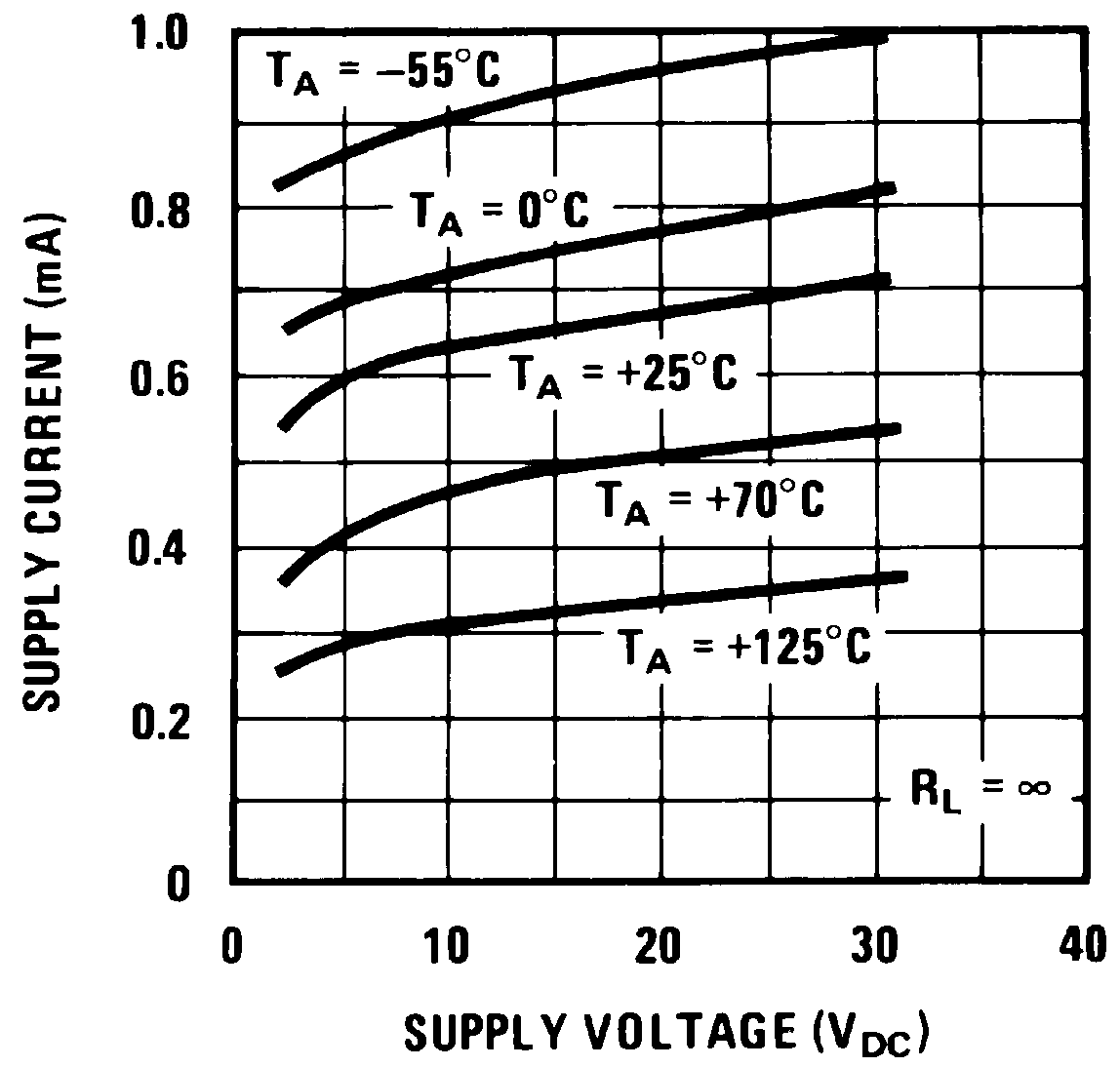Figure 1. Supply Current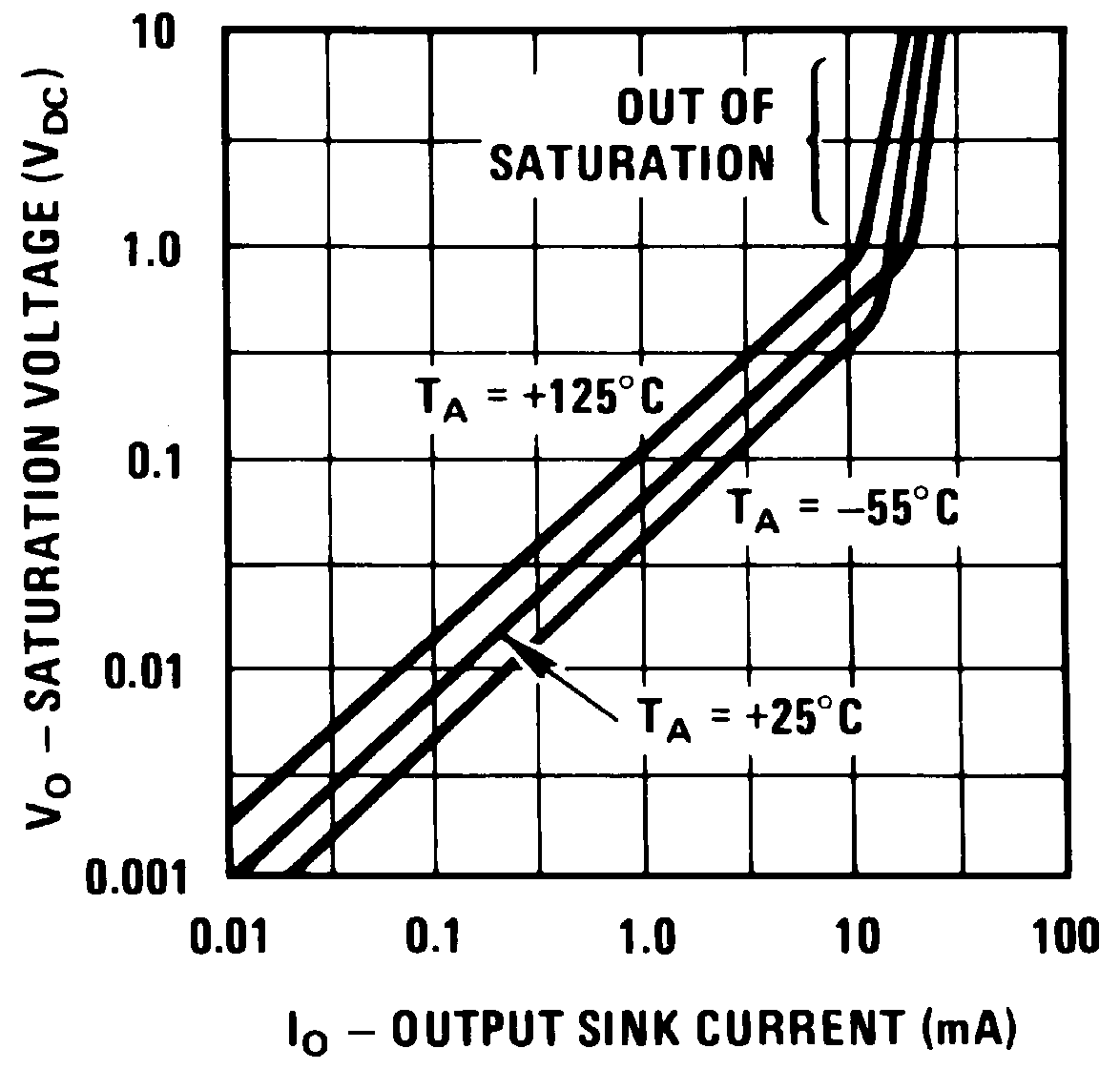Figure 3. Output Saturation Voltage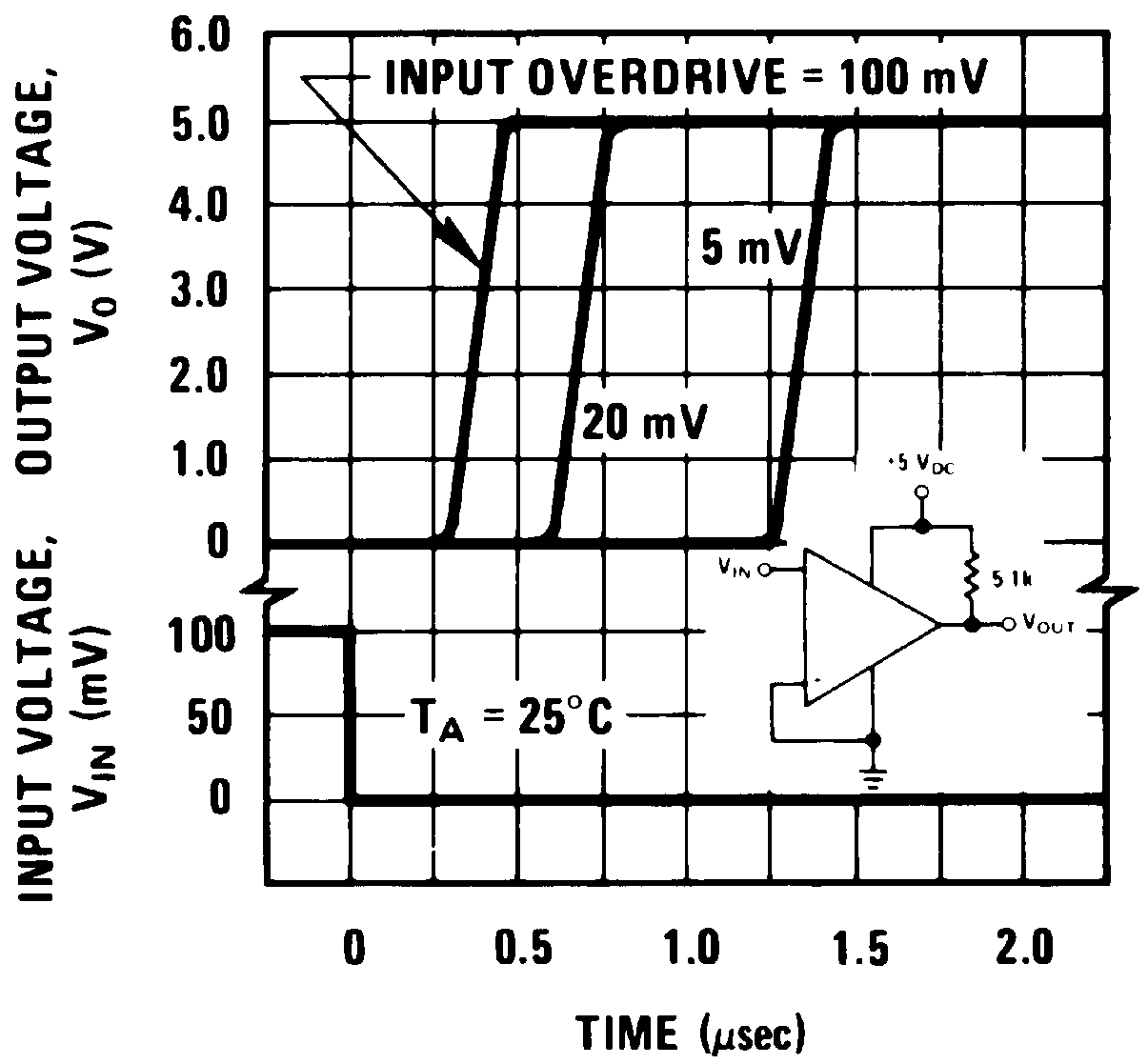Figure 5. Response Time for Various Input Overdrives –
Positive Transition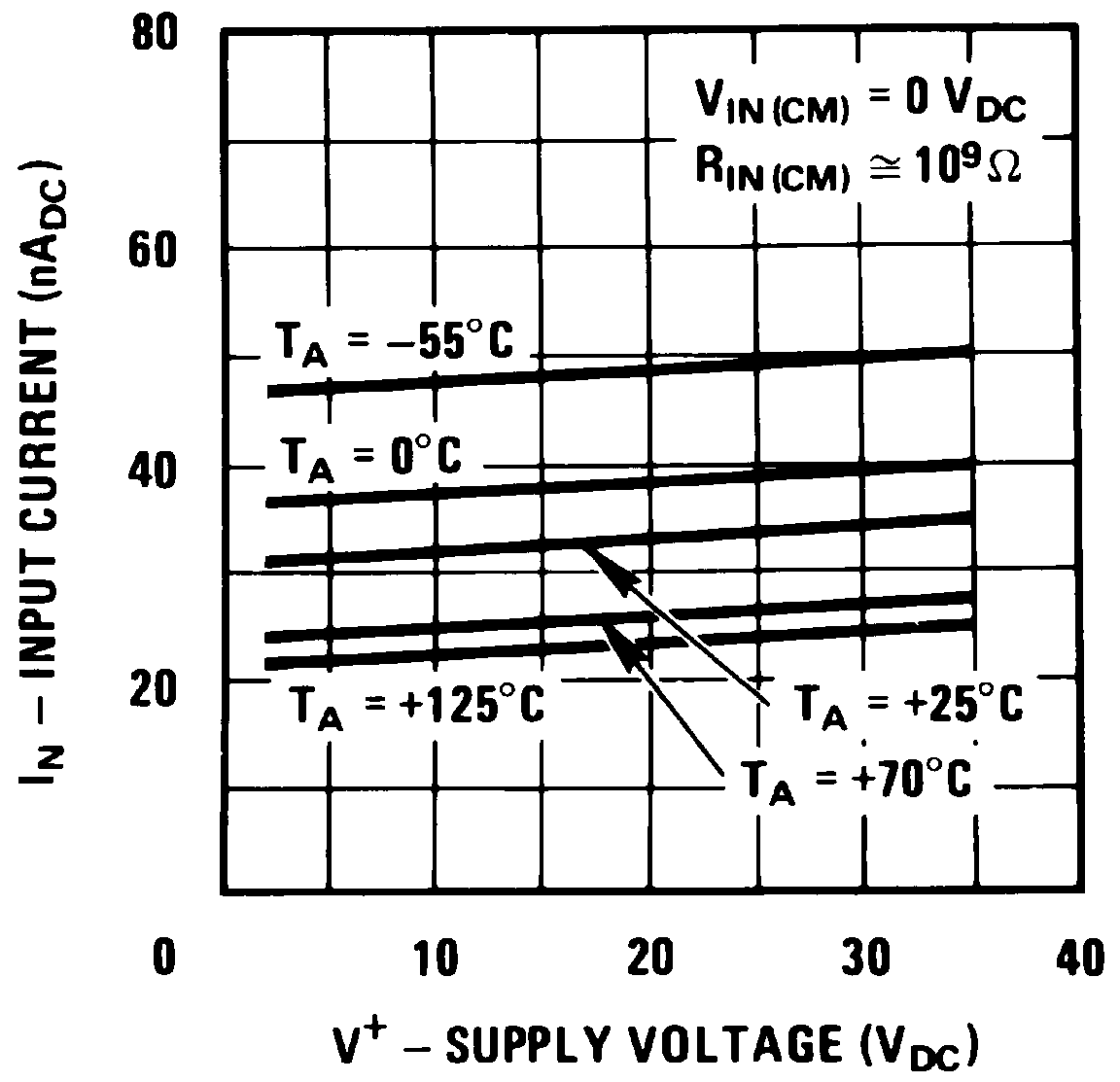Figure 2. Input CurrentFigure 4. Response Time for Various Input Overdrives –
Negative Transition

#### 6.7.2 LM2901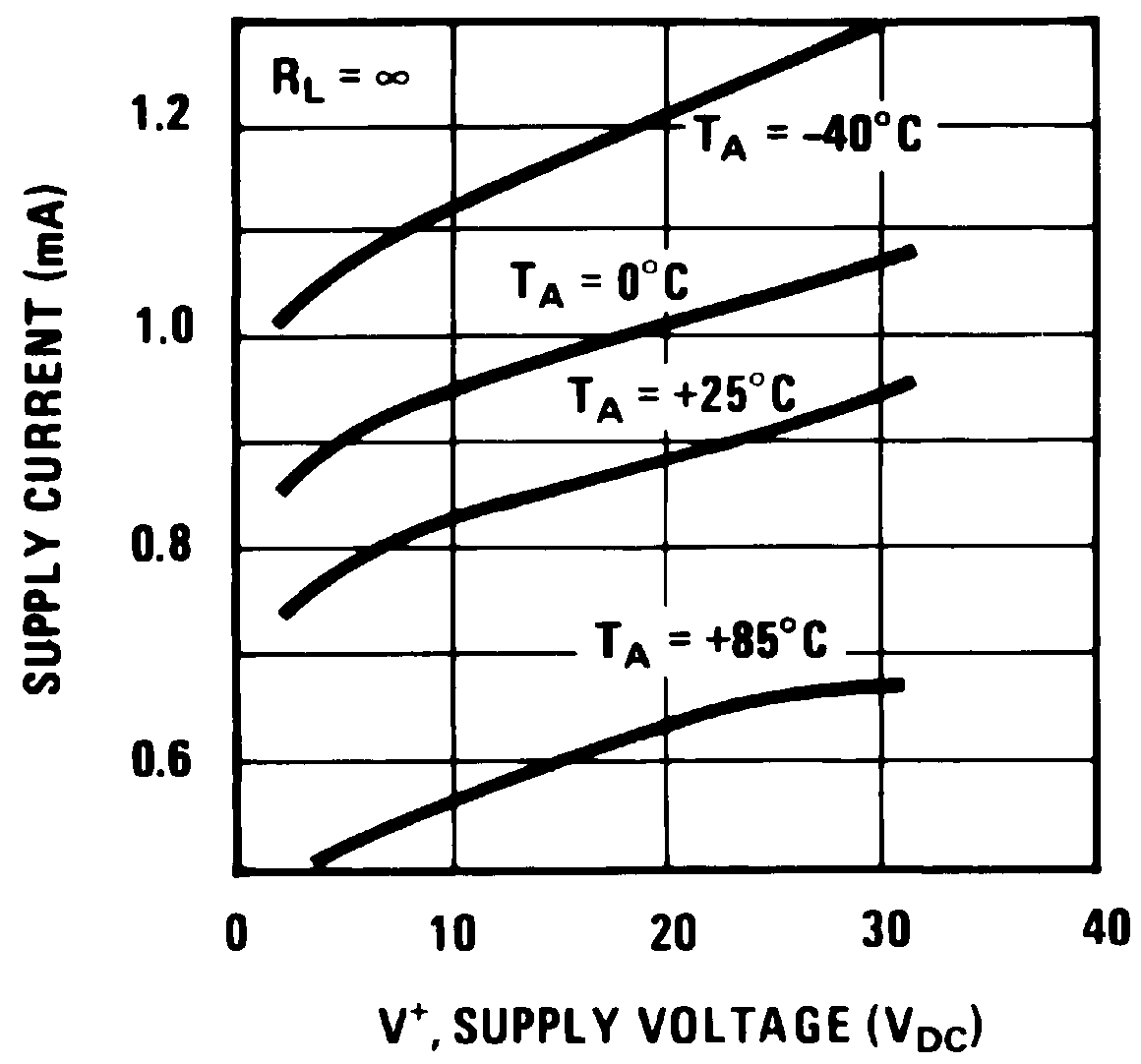Figure 6. Supply Current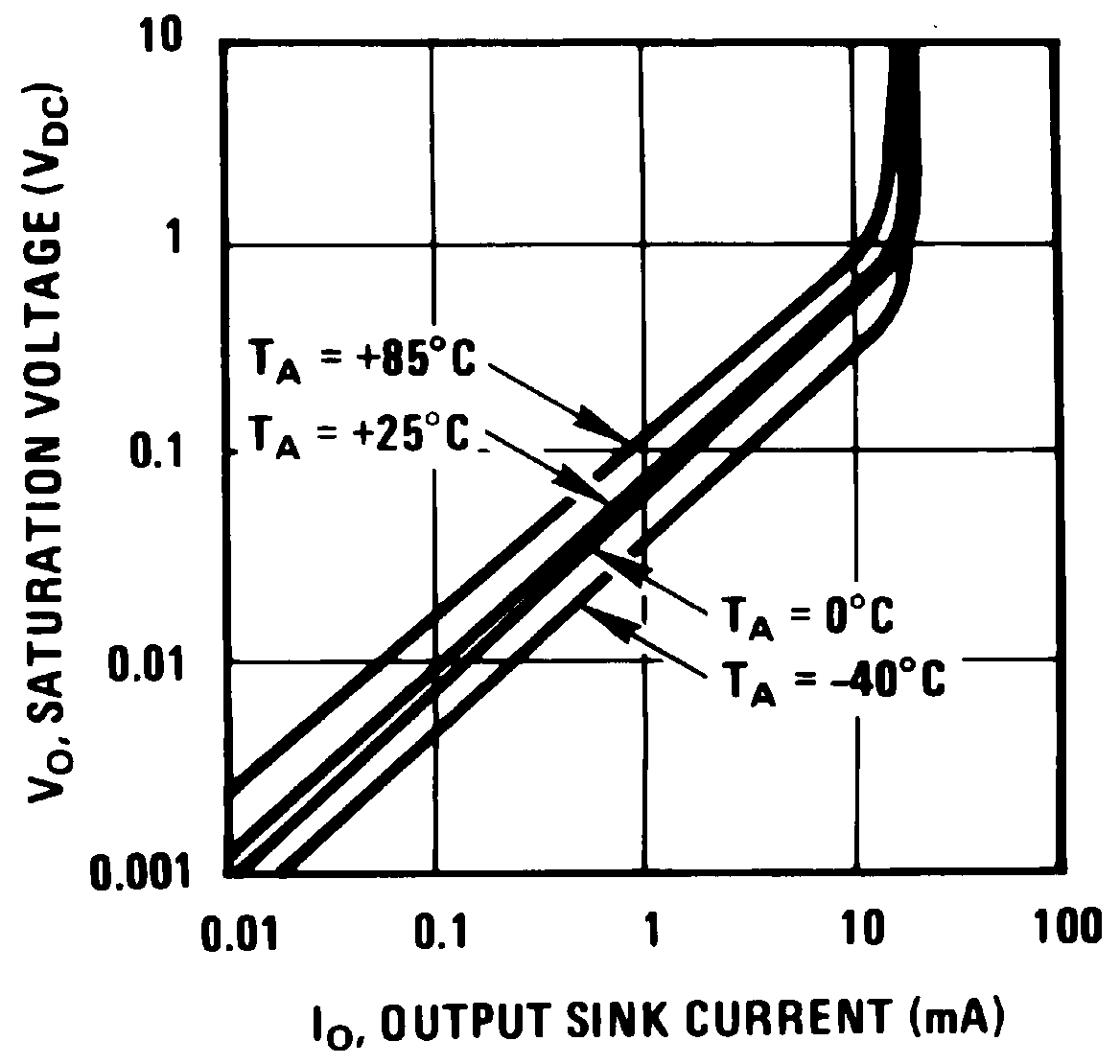Figure 8. Output Saturation Voltage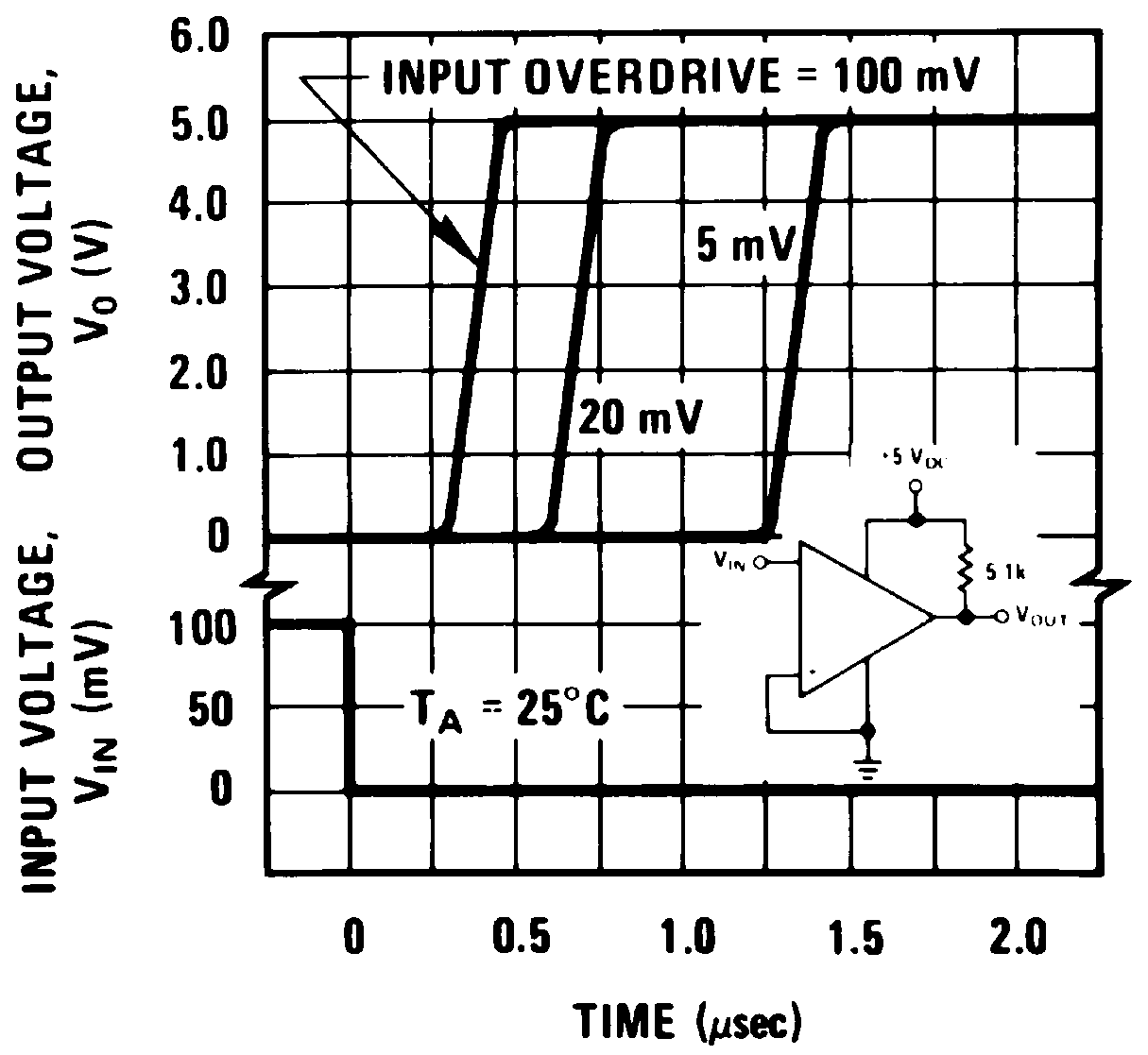Figure 10. Response Time for Various Input Overdrives –
Positive Transition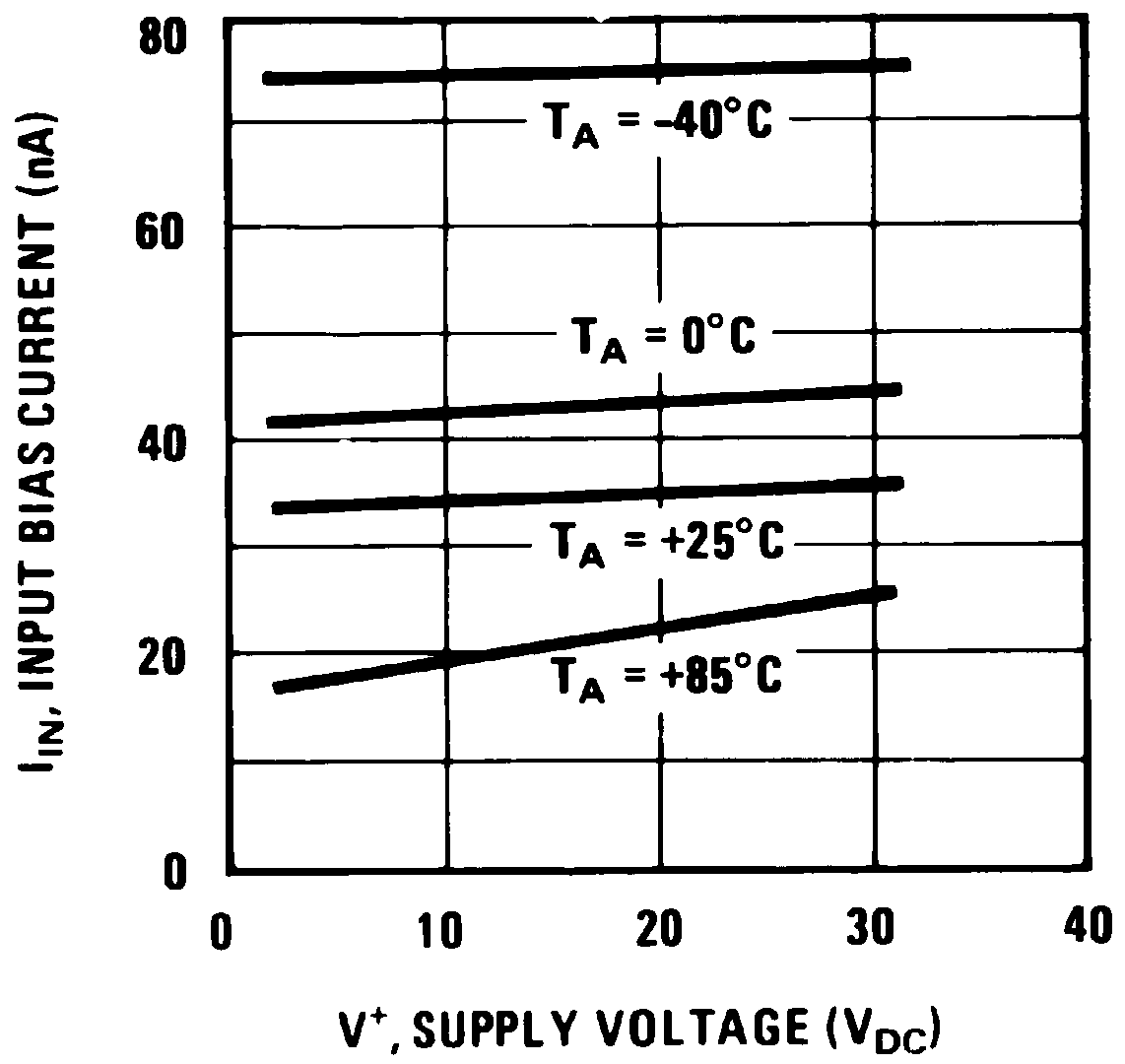Figure 7. Input Current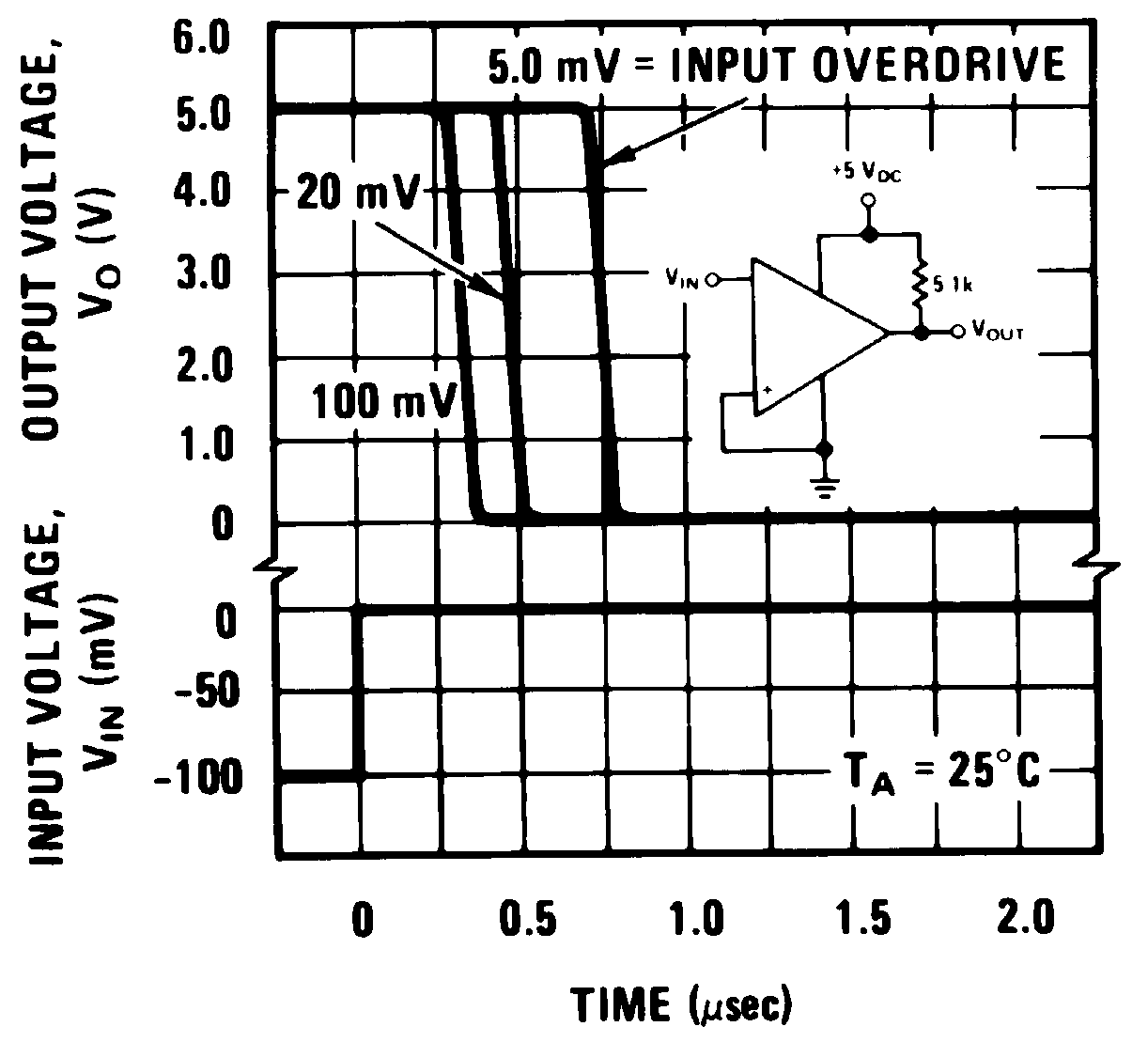Figure 9. Response Time for Various Input Overdrives –
Negative Transition